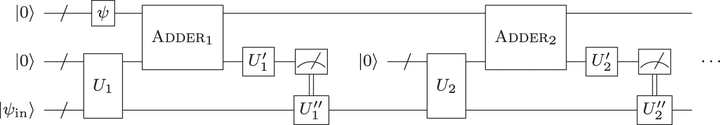# Approximate Quantum Fourier Transform with $O(n\log(n))$ T gates### Abstract

The ability to implement the Quantum Fourier Transform (QFT) efficiently on a quantum computer facilitates the advantages offered by a variety of fundamental quantum algorithms, such as those for integer factoring, computing discrete logarithm over Abelian groups, solving systems of linear equations, and phase estimation, to name a few. The standard fault-tolerant implementation of an $n$-qubit unitary QFT approximates the desired transformation by removing small-angle controlled rotations and synthesizing the remaining ones into Clifford+$\mathrm{T}$ gates, incurring the $\mathrm{T}$-count complexity of $O(n\log^2(n))$. In this paper, we show how to obtain approximate QFT with the $\mathrm{T}$-count of $O(n\log(n))$. Our approach relies on quantum circuits with measurements and feedforward, and on reusing a special quantum state that induces the phase gradient transformation. We report asymptotic analysis as well as concrete circuits, demonstrating significant advantages in both theory and practice.

Type
Publication
npj Quantum Information 6, 26 (2020)##### Yuan Su
###### Research Scientist

I work on quantum algorithms for simulating Hamiltonian dynamics. I am particularly interested in the design, analysis, implementation, and application of quantum simulation.